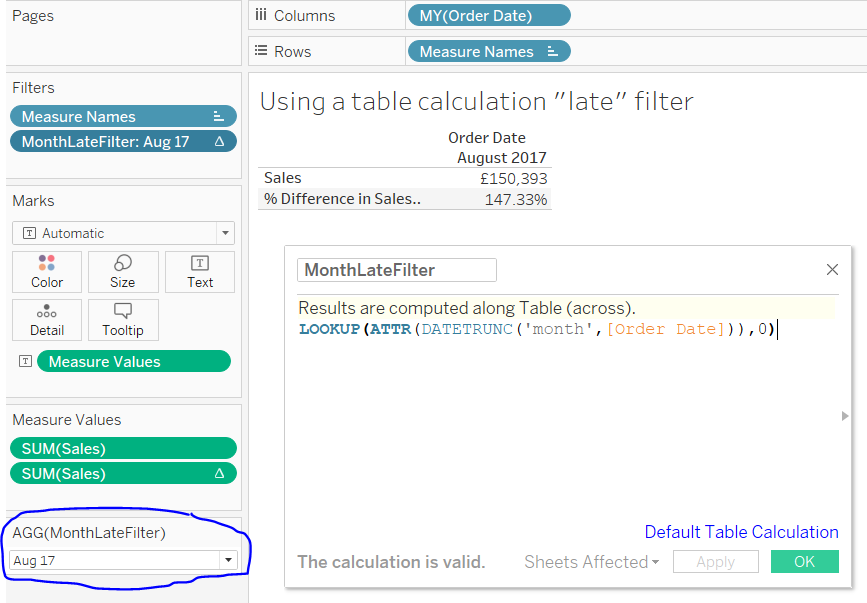This type of question, ‘How do I show prior month when using a month filter?’, ‘My Year on Year is blank when I filter?’,  appears A LOT on the Tableau forums.

There are two main ways to show the prior period / keep a previous value for prior period comparison.

1. Parameter

• Create a parameter to enable selection of a month/year
• Create a calculated field to use this parameter to filter the data – for example DATETRUNC(‘month’,DATEADD(‘month’,1,[DateField])) >= [MonthParameter] OR DATETRUNC(‘month’,[DateField]) = [MonthParameter]
• Drag the calculated field to the filter shelf and set to True

2. Late Filter

This is used more when wanting to show a MoM change or a YoY change. A standard filter removes the prior value from the data so the MoM or YoY is blank.

One of the last things to calculate in Tableau, based on Tableau’s Order of Operations, are table calculations. Therefore if the Month on Month (or Year on Year) is calculated first, then a table calculation filter is applied the MoM/YoY calculation will remain good.

• Create the calculation to get the value for the prior period: LOOKUP(SUM([Sales),-1)
• Create a calculated field to be the month filter. This has to be a table calculation to happen after the prior period is calculated. LOOKUP(ATTR([DateField]),0)
• Drag the month calculated field to the filter shelf and Show Filter.

Both of these options have pros and cons, which is better for you depends on the specific requirements. For example a parameter filter doesn’t allow multi-select and can’t ‘Show Relevant Values’. A table calculation filter can only apply to a single worksheet so provides limited use in a dashboard if intended to drive multiple worksheets.

Examples

Both examples are using the Sample Superstore EU data source. Both are set up the same, using a Quick Table Calculation doing Percent Difference and using the default settings. We’re returning the month on month change.

Tableau Parameter Filter example

• Create a parameter, MonthParameter, based on the Order Date field. Format to month and year: mmm yy.
• Create a calculated field, MonthFilter, to use that parameter. This is where we’re enabling the parameter to be used as a filter. Note the usage of DATETRUNC. This forces any date selected in a month to be the 1st of the month therefore Order Dates of 15 Aug would be converted to 1 Aug.

[MonthFilter]

AND DATETRUNC(‘month’,[Order Date]) <= DATETRUNC(‘month’,[MonthParameter])

• Drag the MonthFilter to the filter shelf and set to True.Tableau Lookup Late Filter

• Create a calculated field which will be used as the month filter

[MonthLateFilter]

LOOKUP(ATTR(DATETRUNC(‘month’,[Order Date])),0)

• I used DATETRUNC so all order dates within a month are set to the same exact date. Table calculations have to be an aggregation, therefore we have to use ATTR as it’s most appropriate for this use case. However this will only work if all the dates for a month/year are the same. Different dates within a month would return a *, which is no good for the filter.
• Set the Default Properties – Date Format to Custom mmm yy
• Drag the field from Measures to the Filter shelf.The workbook with these examples is available on Tableau Public here: https://public.tableau.com/views/IncludePriorPeriodExamples/ShowPriorPeriod